# Hilbert-Schmidt operator

(Redirected from Hilbert–Schmidt operator)
Jump to: navigation, search

An operatoracting on a Hilbert spacesuch that for any orthonormal basisinthe following condition is met: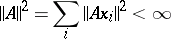(however, this need be true for some basis only). A Hilbert–Schmidt operator is a compact operator for which the conditionapplies to its-numbersand its eigen values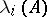; here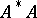is a trace-class operator (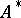is the adjoint ofand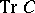is the trace of an operator). The set of all Hilbert–Schmidt operators on a fixed spaceforms a Hilbert space with scalar product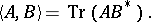Ifis the resolvent ofand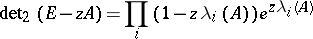is its regularized characteristic determinant, then the Carleman inequalityholds.

A typical representative of a Hilbert–Schmidt operator is a Hilbert–Schmidt integral operator (which explains the origin of the name).

#### Comments

The-numbers or singular values ofare the (positive) eigen values of the self-adjoint operator. Instead of Hilbert–Schmidt operator one also says "of Hilbert–Schmidt class operatorHilbert–Schmidt class" . A bounded operatoron a Hilbert space is said to be of trace class if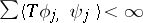for arbitrary complete orthonormal systems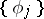,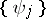. Equivalently,is of trace class if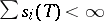. The trace of such an operator is defined as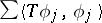, whereis any orthonormal basis. The product of two Hilbert–Schmidt operators is of trace class and the converse is also true.

The norm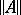in the above article is not the usual operator norm ofbut its Hilbert–Schmidt norm.